SLVSDT1C July   2017  – June 2020

PRODUCTION DATA.

1. Features
2. Applications
3. Description
1.     Device Images
4. Revision History
5. Pin Configuration and Functions
6. Specifications
7. Detailed Description
1. 7.1 Overview
2. 7.2 Functional Block Diagram
3. 7.3 Feature Description
4. 7.4 Device Functional Modes
8. Application and Implementation
1. 8.1 Application Information
2. 8.2 Typical Application
1. 8.2.1 Design Requirements
2. 8.2.2 Detailed Design Procedure
3. 8.2.3 Application Curves
3. 8.3 System Examples
9. Power Supply Recommendations
10. 10Layout
11. 11Device and Documentation Support
12. 12Mechanical, Packaging, and Orderable Information

• MOP|24

8.2.3 Application Curves

VIN= 3.6 V, VOUT = 1.8V (R1 / R2 = 240 kΩ / 120 kΩ), TA = 25°C, MODE = Low, (unless otherwise noted)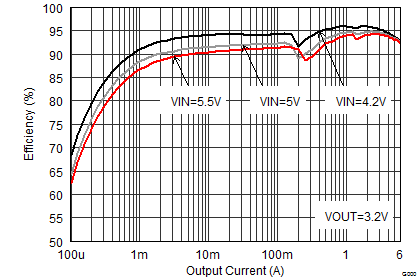VOUT = 3.2 V
Figure 10. Efficiency vs Output Current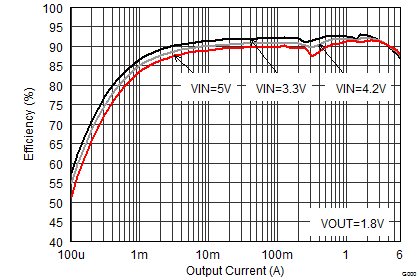VOUT = 1.8 V
Figure 12. Efficiency vs Output Current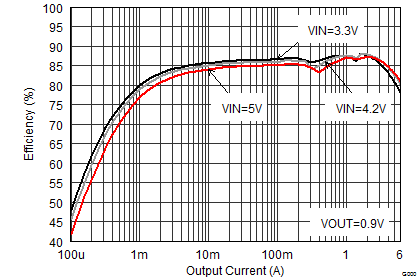VOUT = 0.9 V
Figure 14. Efficiency vs Output Current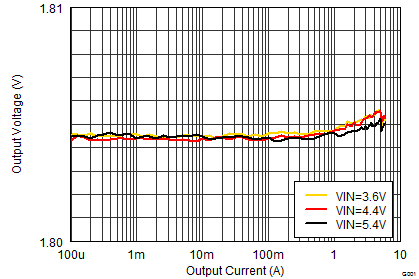MODE = High
Figure 16. Output Voltage vs Output Current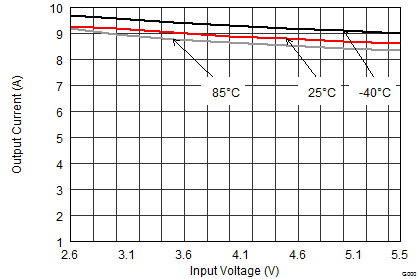VOUT = 0.6 V
Figure 18. Maximum Output Current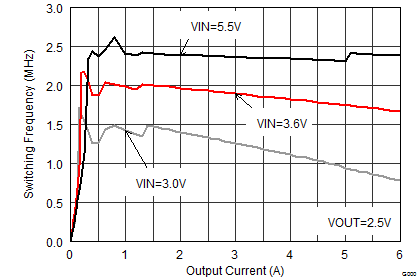VOUT = 2.5 V
Figure 20. Switching Frequency vs Output Current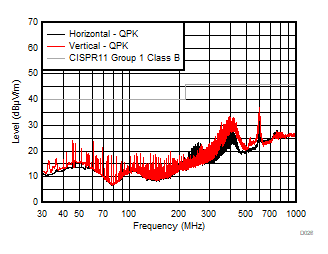VIN = 6 V (battery supply) RLOAD = 0.33 Ω VOUT = 1.8 V EMI test board without filters VSEL Low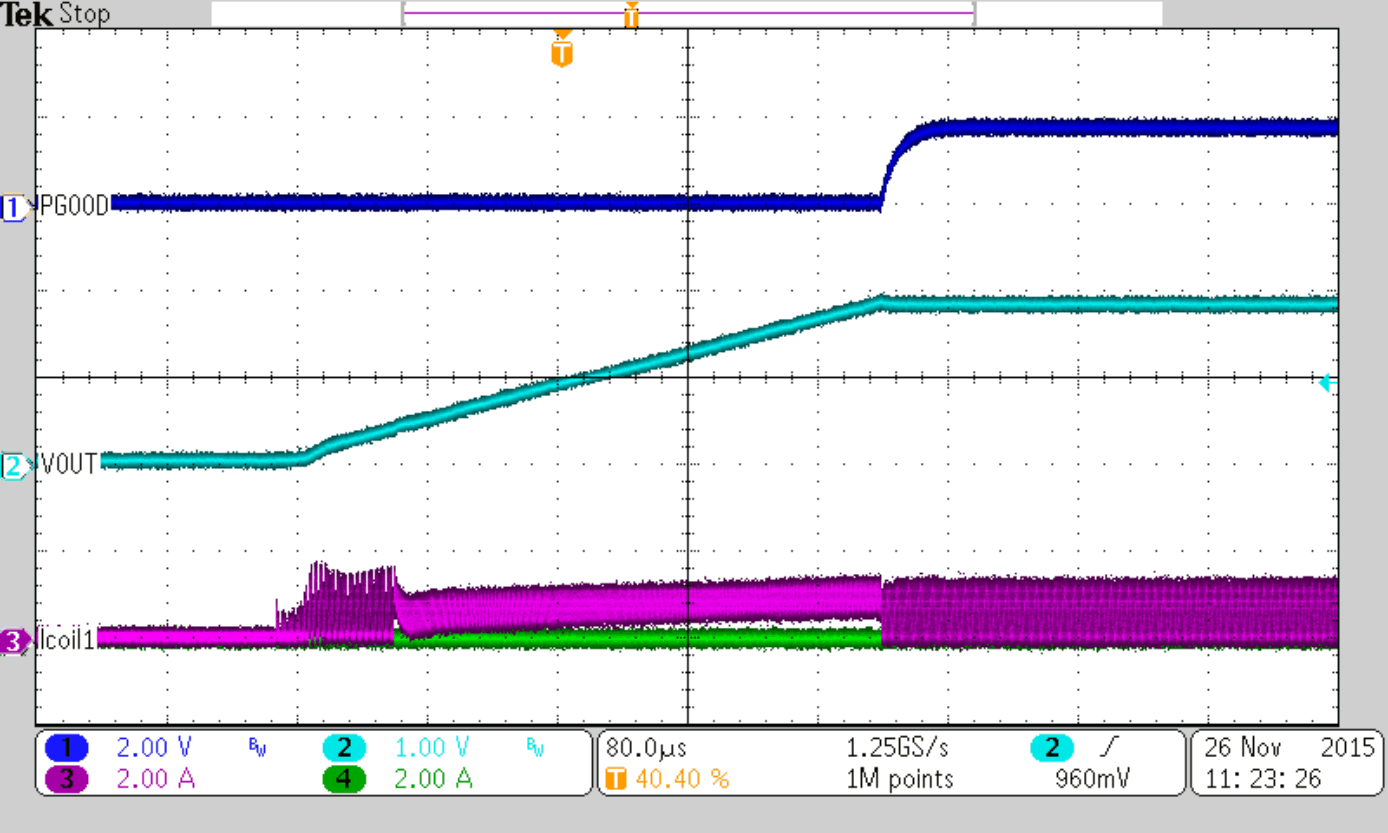VOUT = 1.8 V
Figure 24. Startup into 3.3 Ω resistor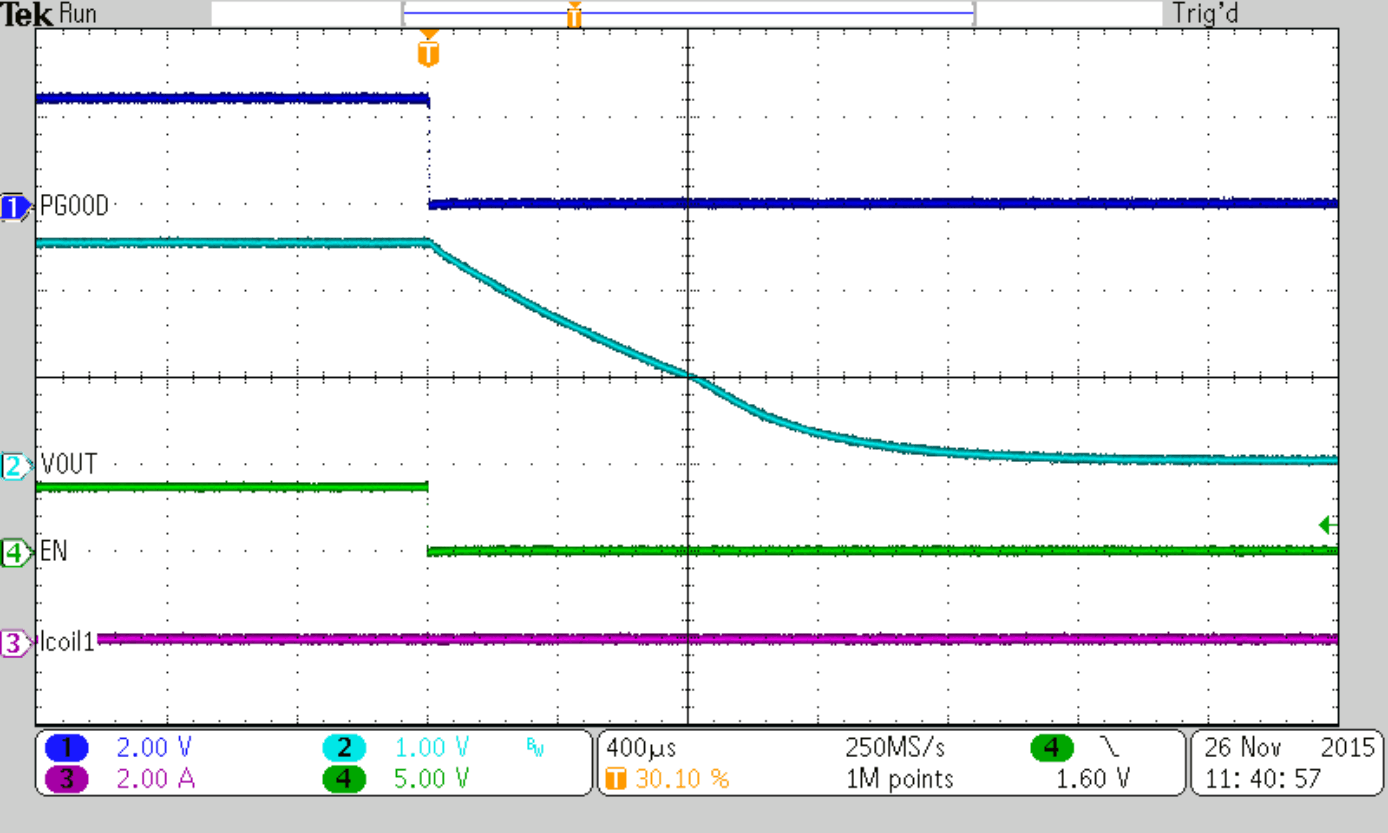VOUT = 2.5 V no load
Figure 26. Output Discharge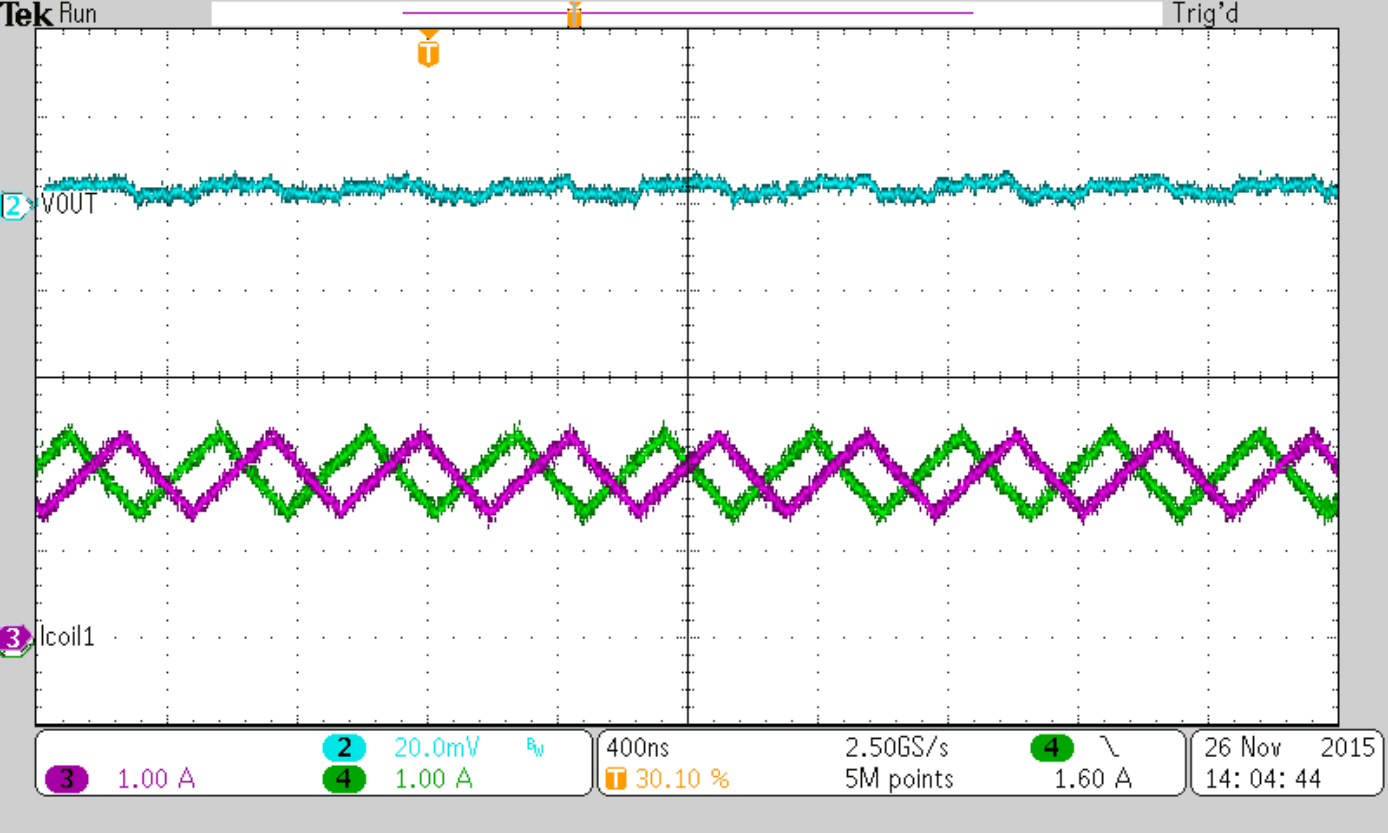Figure 28. Typical Operation PWM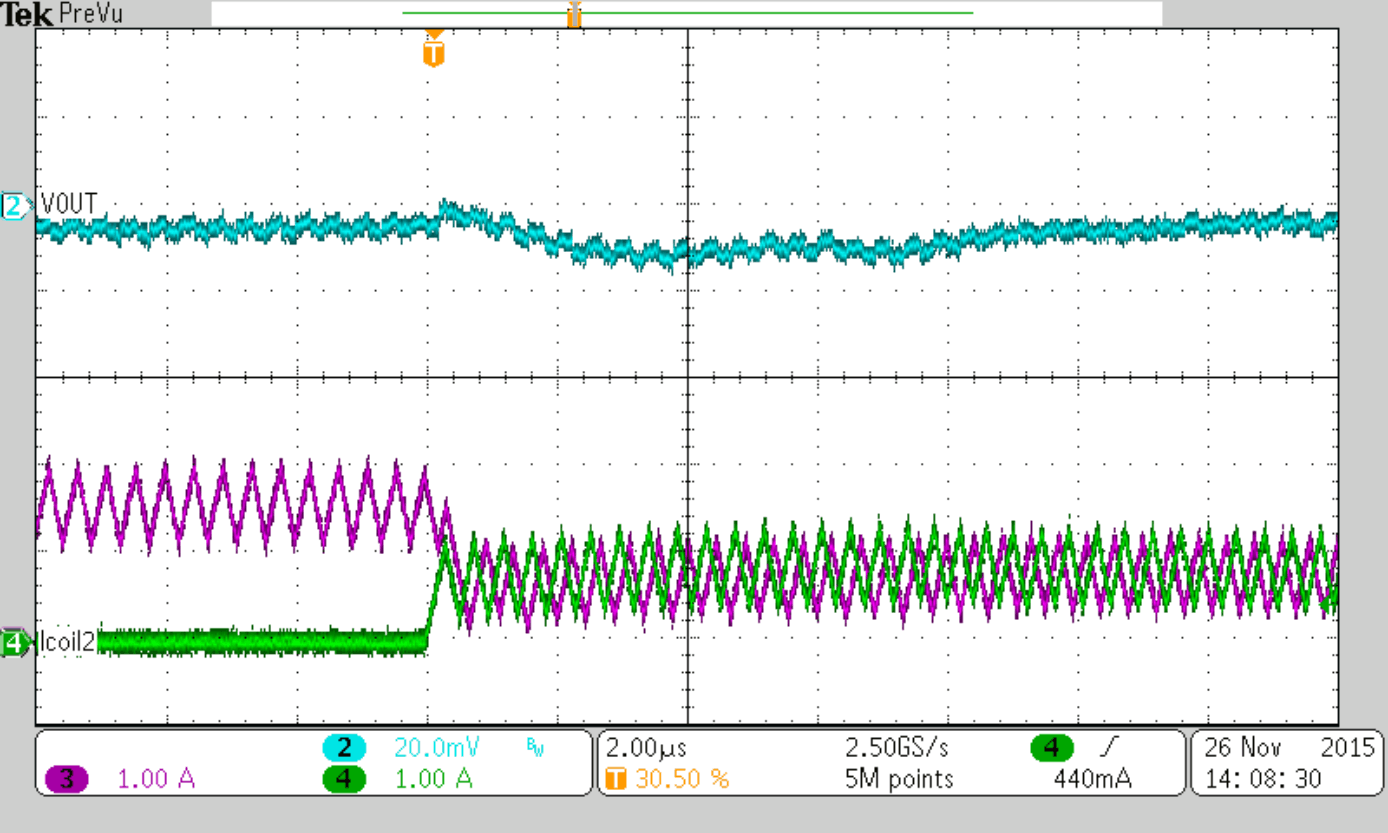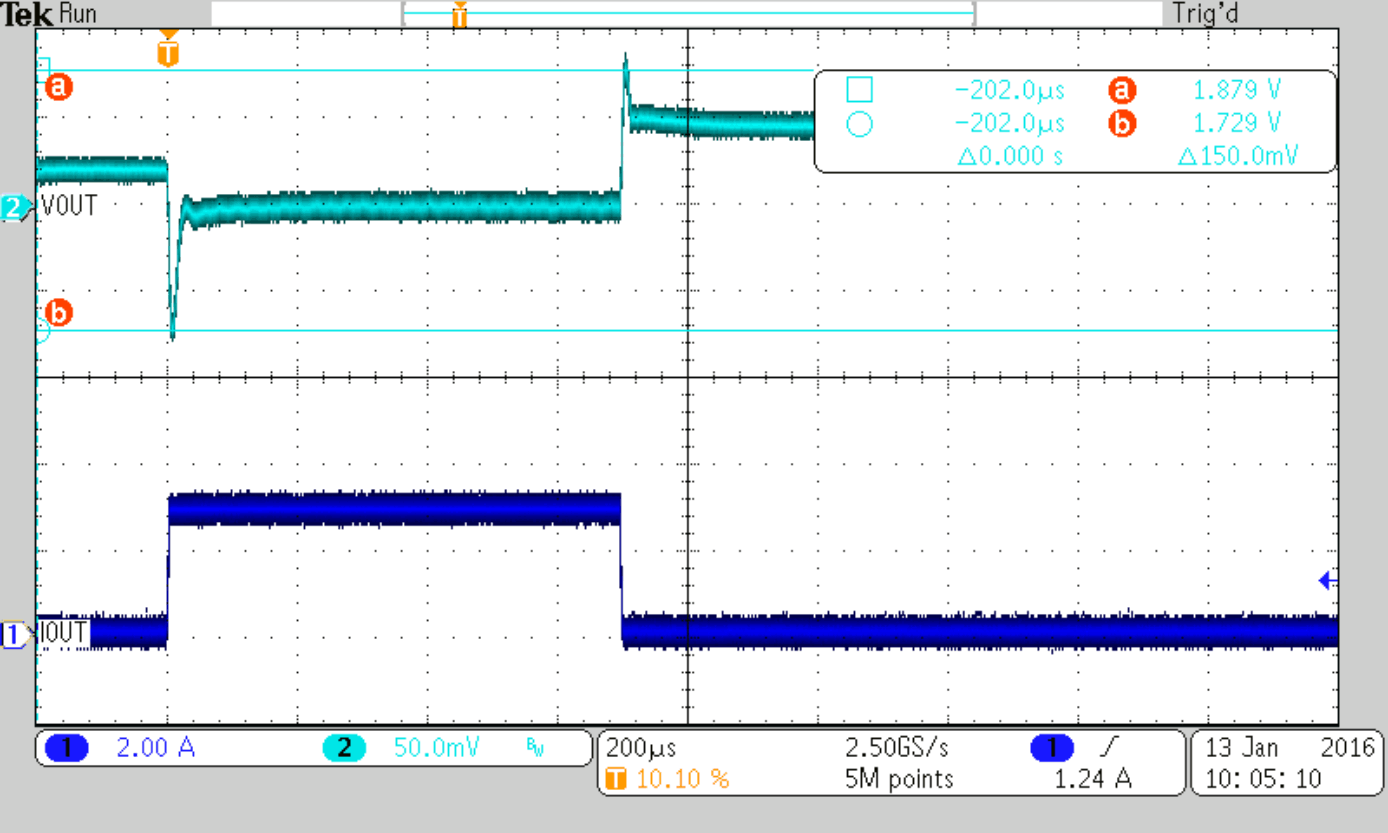Figure 32. Load Transient Response (PSM-PWM),
Load Step 0 to 3 AFigure 34. Load Transient Response (PWM-PWM),
Load Step 3 to 6 A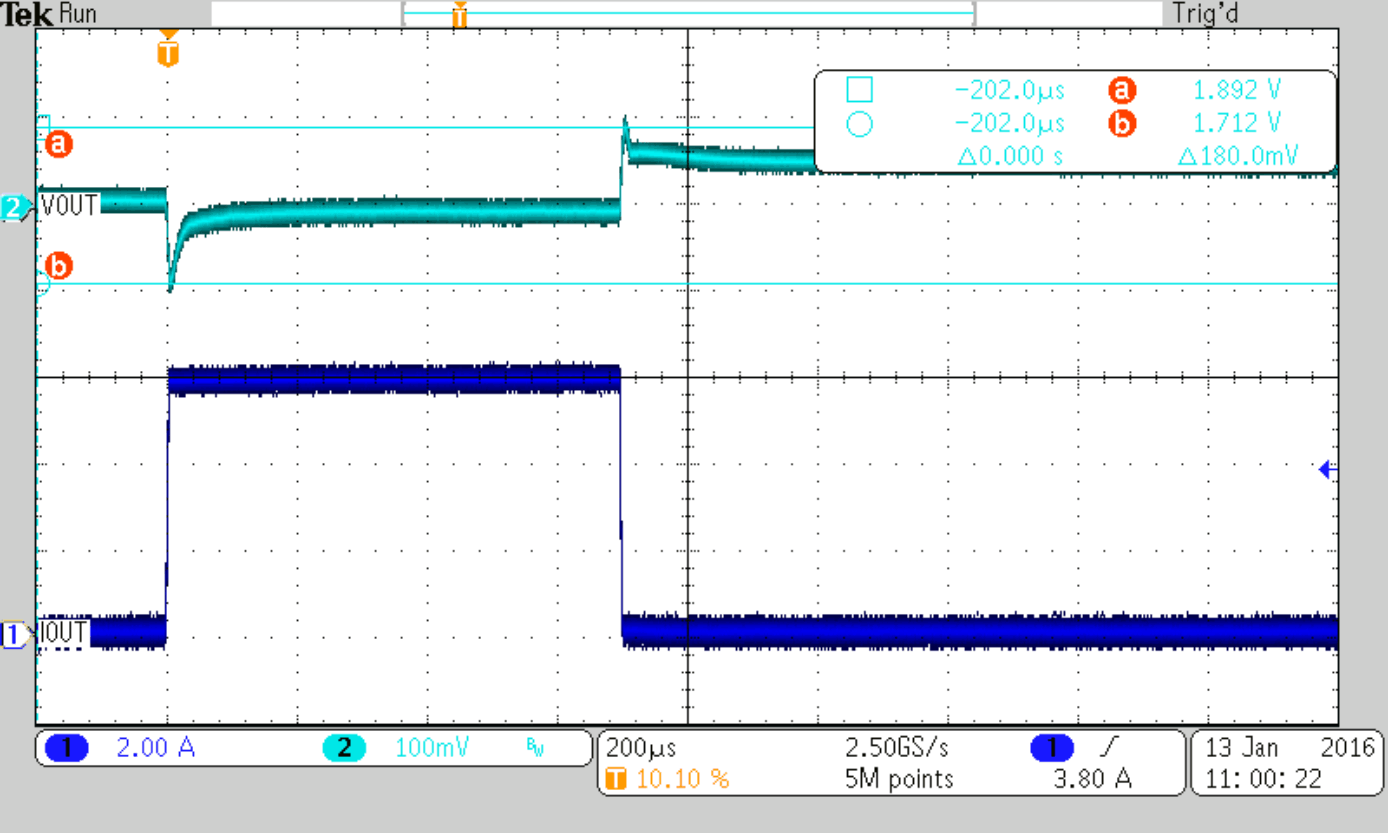Cff = 36 pF (nom)
Figure 36. Load Transient Response (PWM-PWM),
Load Step 0 to 6 A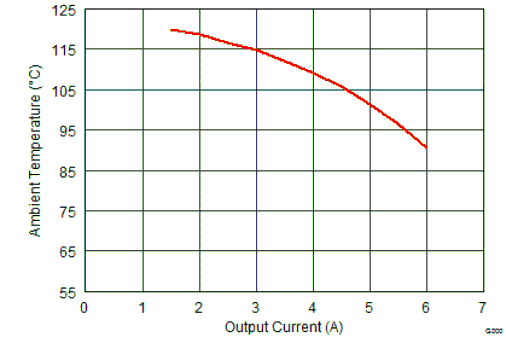VIN = 5 V VOUT = 3.3 V
Figure 38. Maximum Ambient Temperature for TJ=125°C
(TPSM82480 EVM)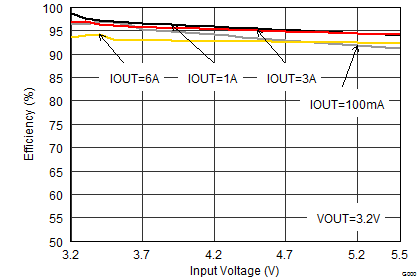VOUT = 3.2 V
Figure 11. Efficiency vs Input Voltage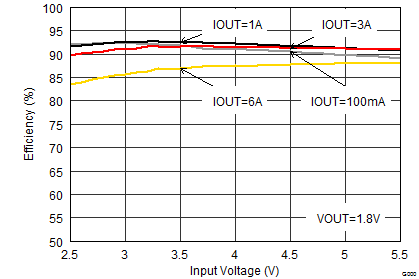VOUT = 1.8 V
Figure 13. Efficiency vs Input Voltage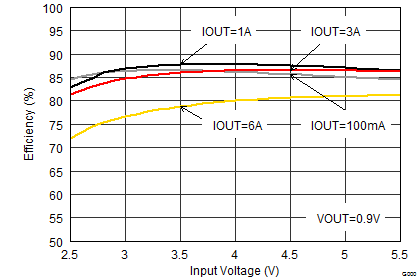VOUT = 0.9 V
Figure 15. Efficiency vs Input Voltage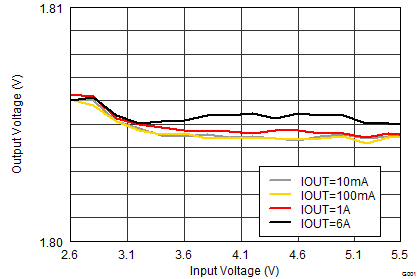MODE = High
Figure 17. Output Voltage vs Input Voltage
(Line Regulation)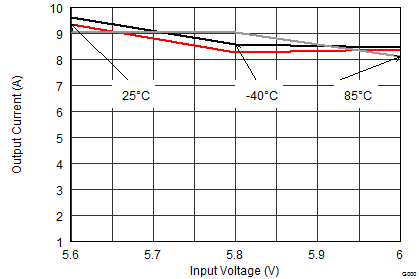VOUT = 5.5 V
Figure 19. Maximum Output Current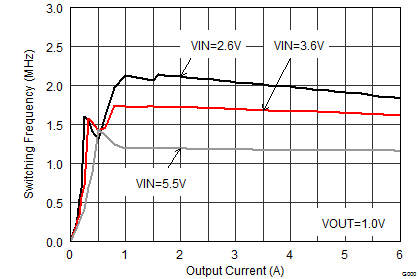VOUT = 1 V
Figure 21. Switching Frequency vs Output Current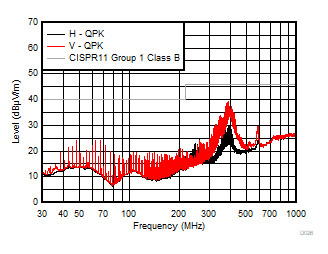VIN = 6 V (battery supply) RLOAD = 0.47 Ω VOUT = 2.5 V EMI test board without filters VSEL High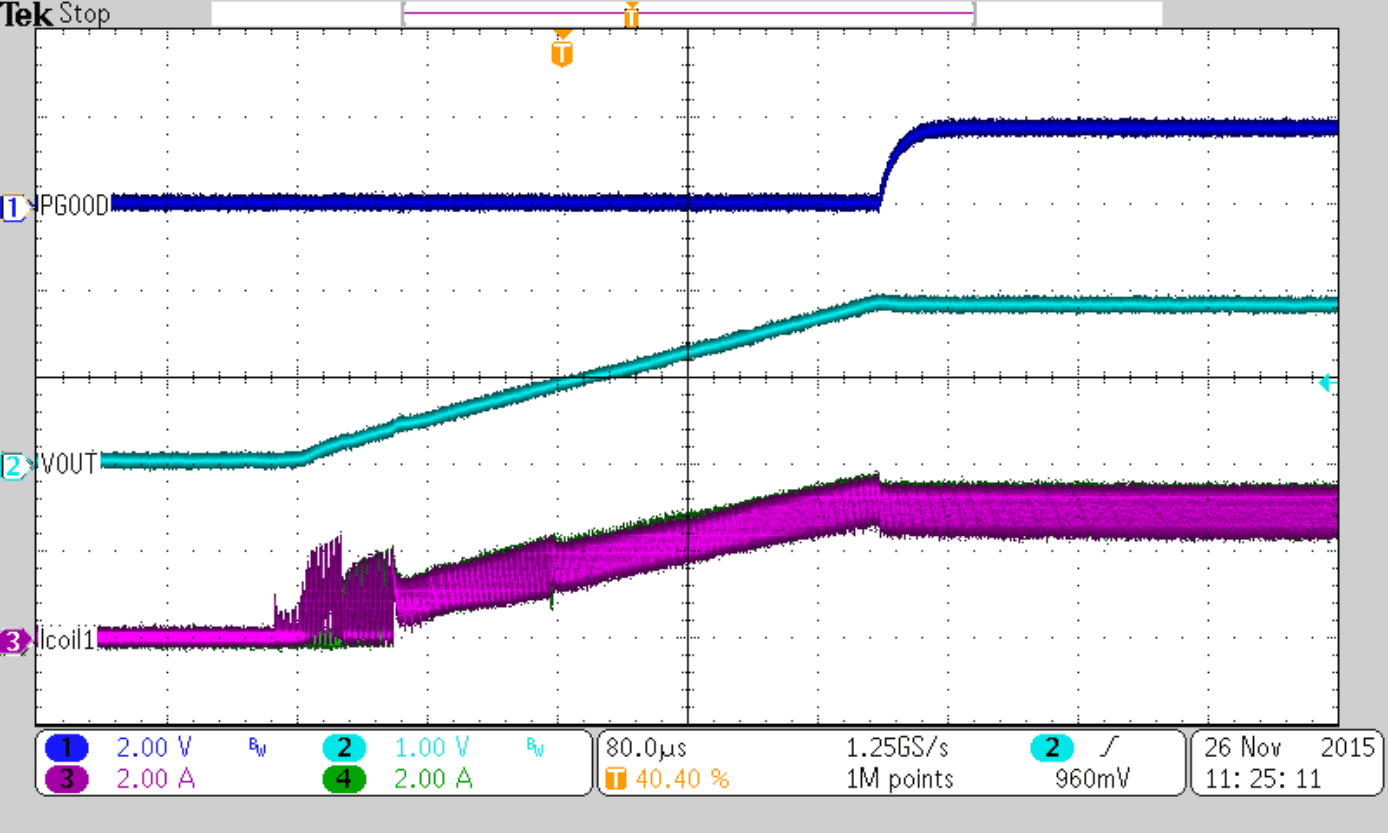VOUT = 1.8 V
Figure 25. Startup into 0.3 Ω resistor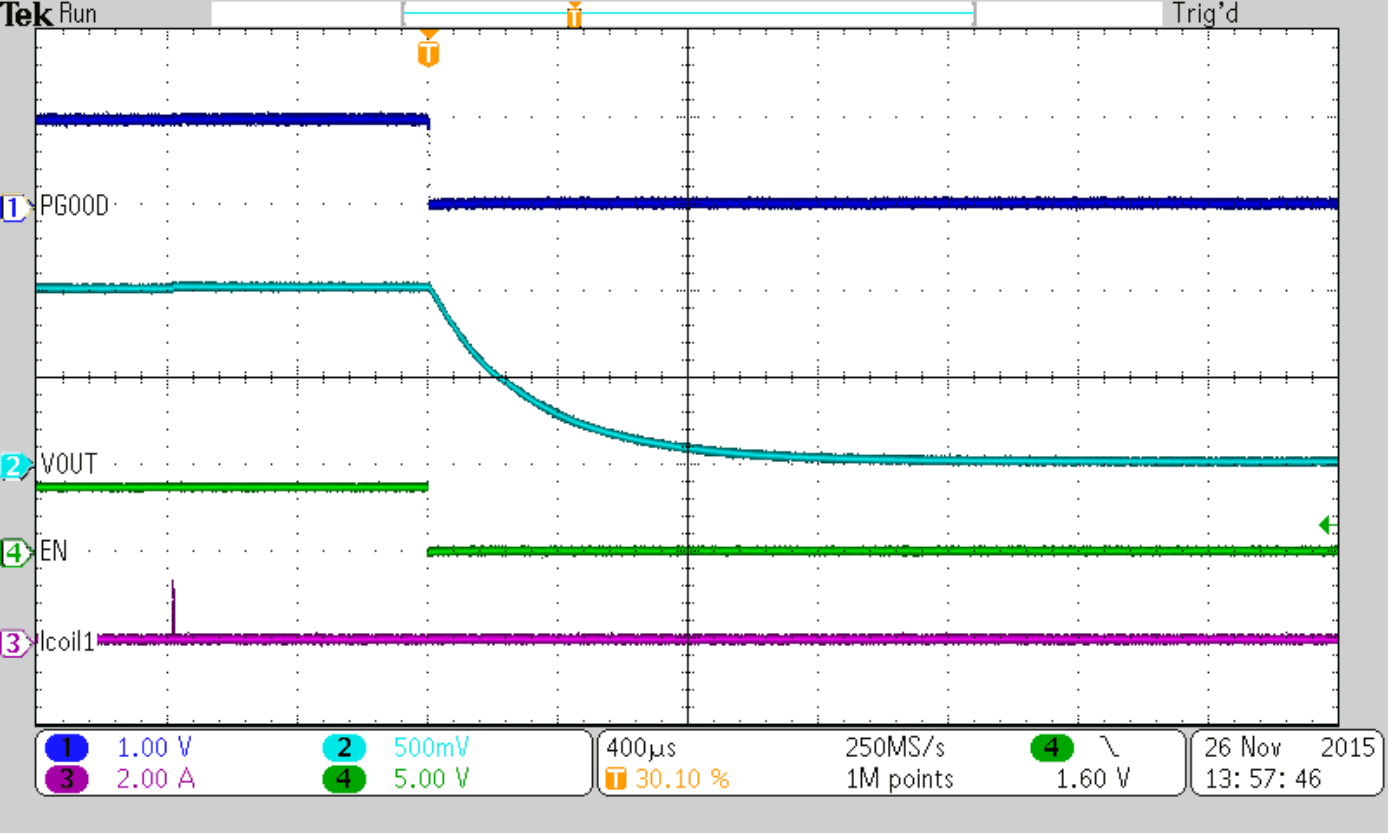VOUT = 1 V no load
Figure 27. Output Discharge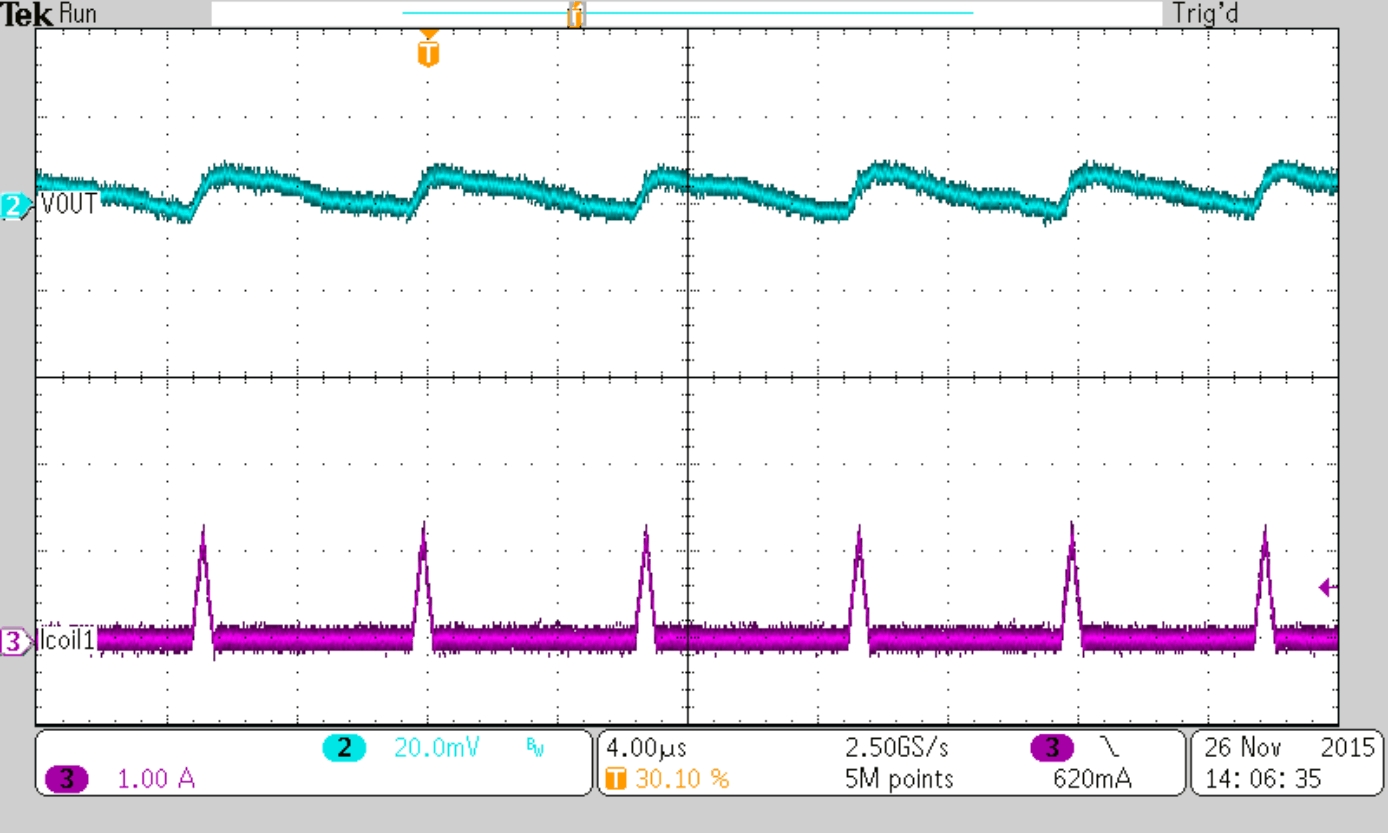IOUT = 50 mA
Figure 29. Typical Operation PSM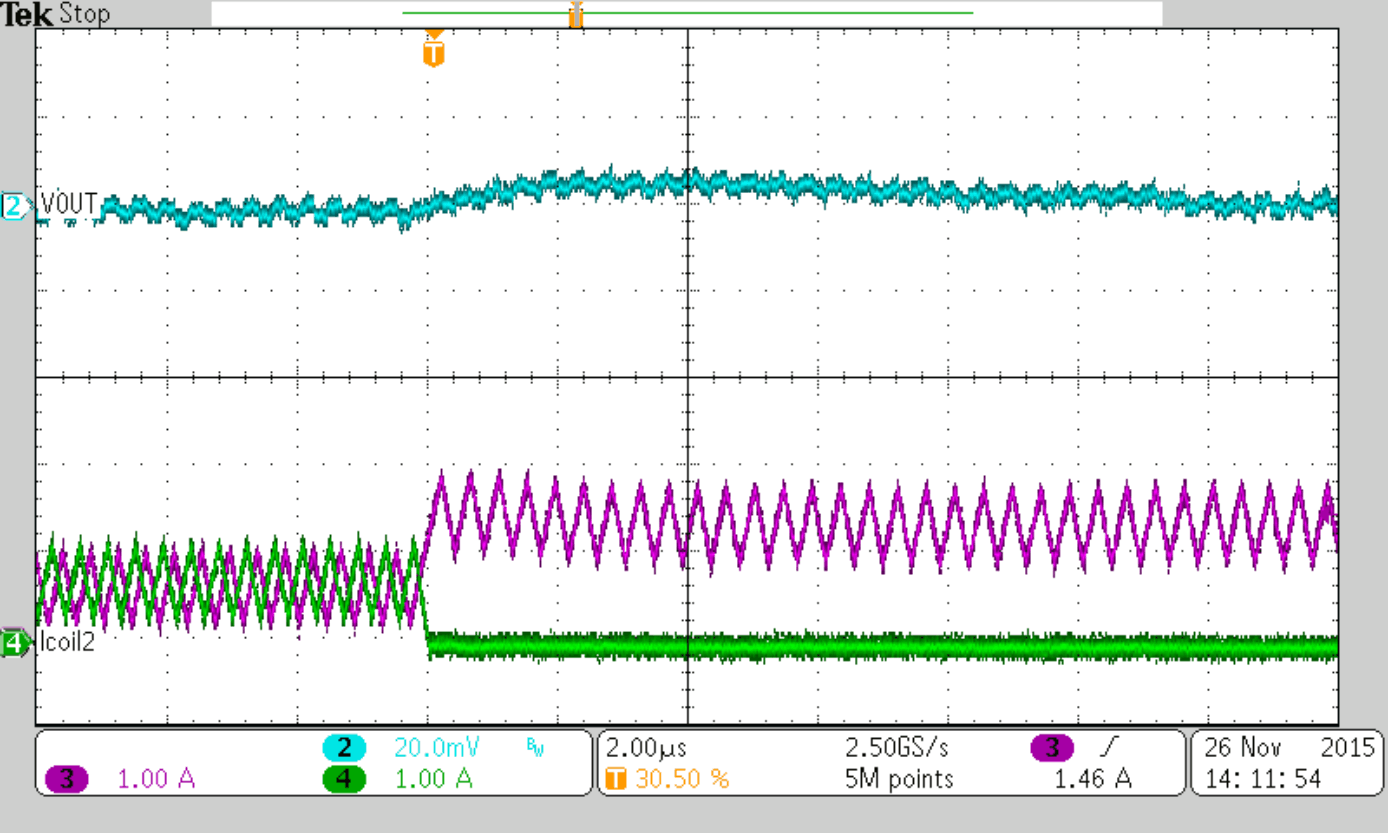Figure 31. Shedding 2nd Phase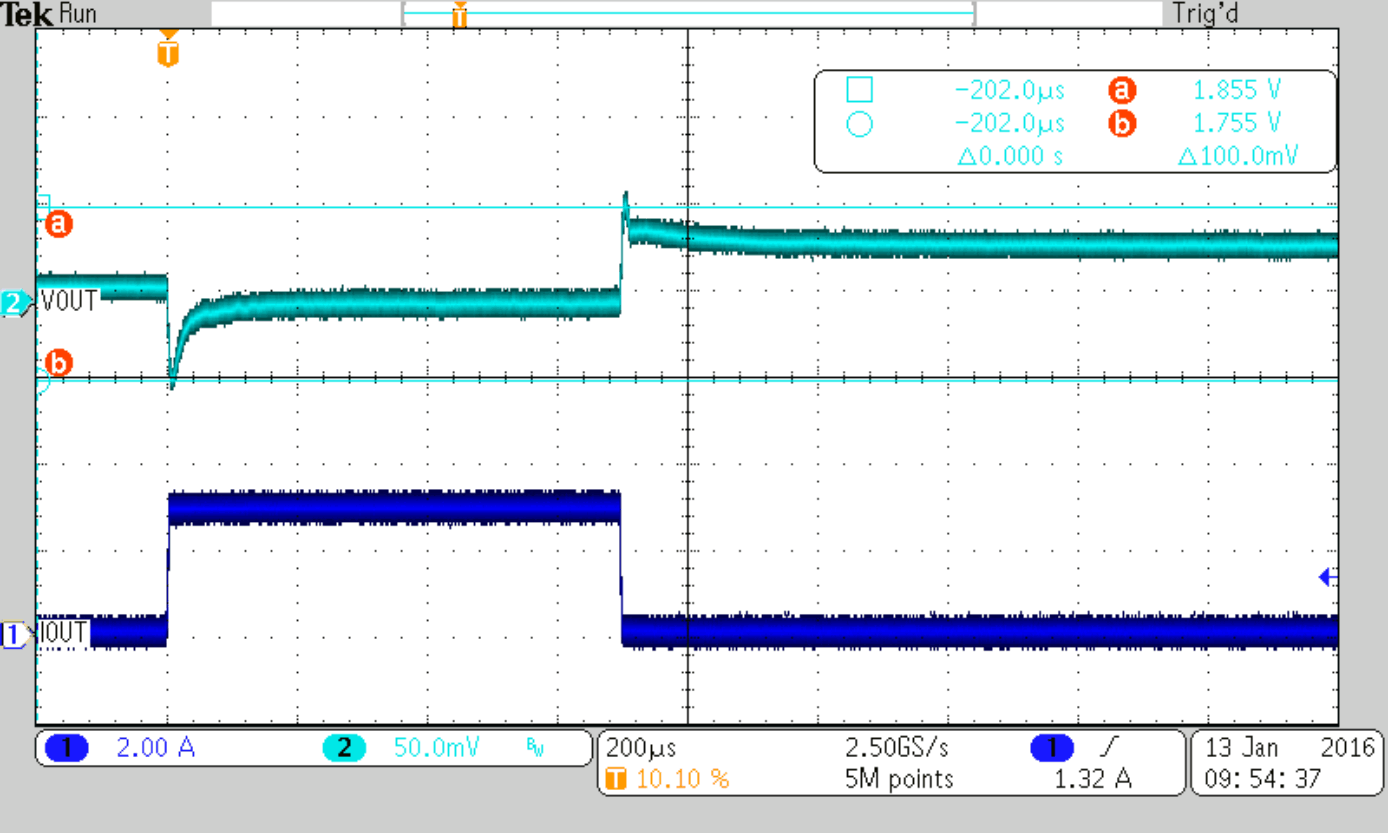Cff = 36 pF (nom)
Figure 33. Load Transient Response (PSM-PWM),
Load Step 0 to 3 A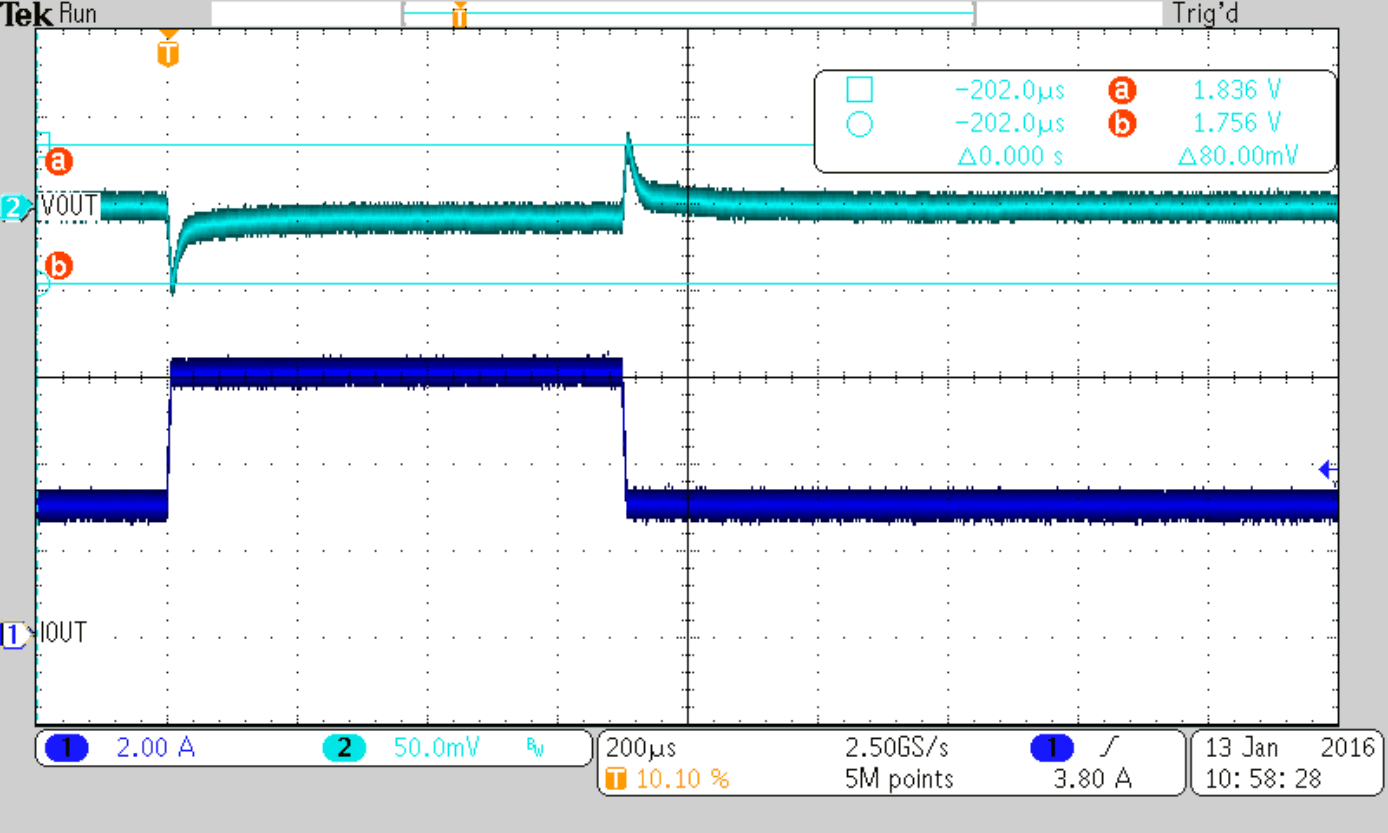Cff = 36 pF (nom)
Figure 35. Load Transient Response (PWM-PWM),
Load Step 3 to 6 A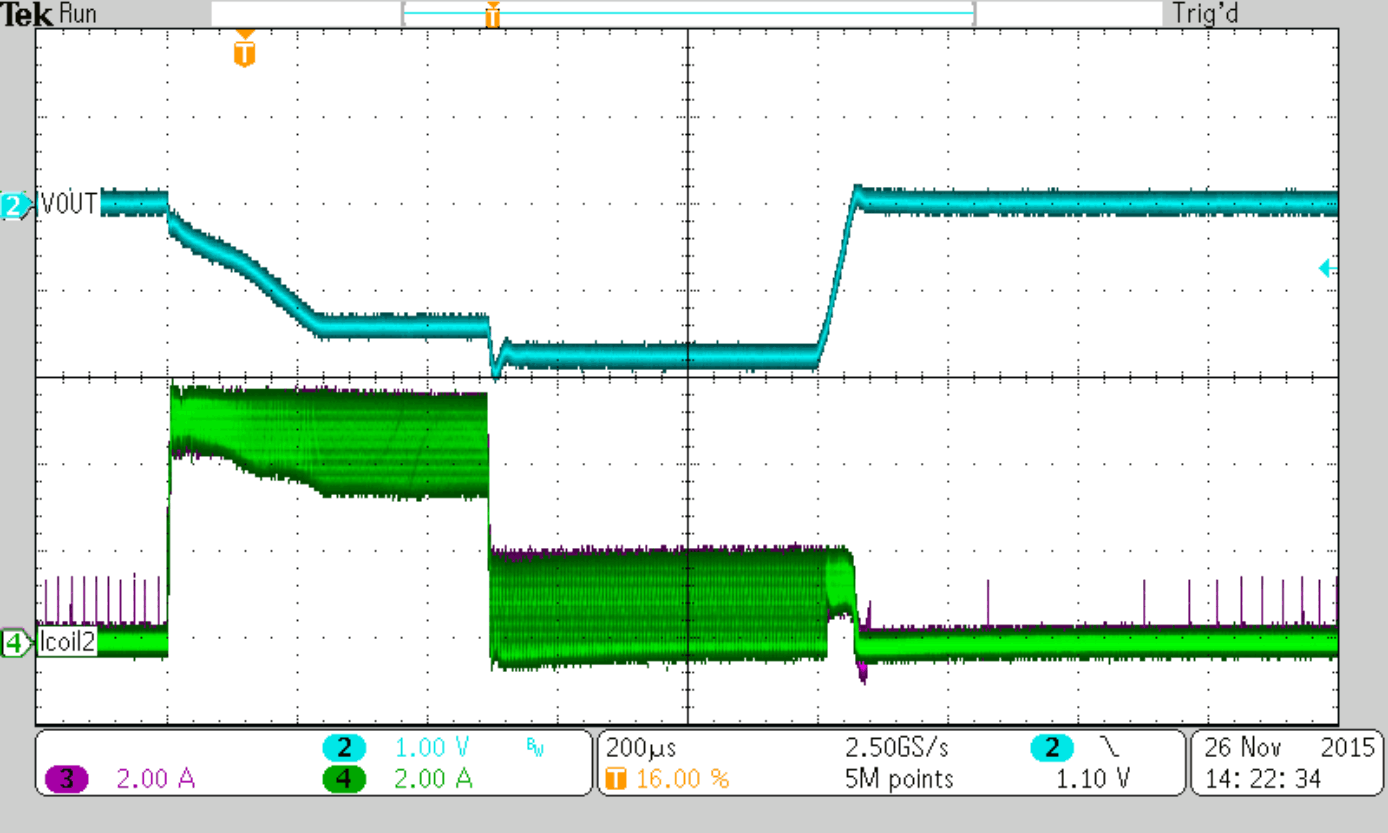IOUT = 10 A
Figure 37. Current Limit Fold-Back at Overload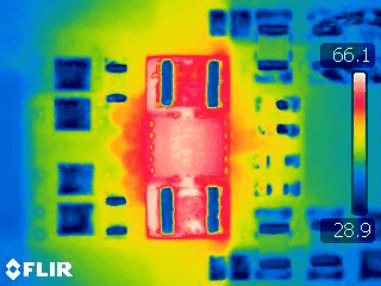VIN = 5 V VOUT = 3.3 V IOUT = 6 A
Figure 39. Device Temperature (TPSM82480 EVM)